# What are the limitations of the amplitude modulation

## Double sideband amplitude modulation

### # OVERVIEW OF THE SECOND MAIN CHAPTER #

After some general explanations of modulation and demodulation, a detailed description of the follows in the second chapter Amplitude modulation and the associated Demodulators. This chapter deals in detail with:

• the description and implementation of the double sideband amplitude modulation (ZSB – AM) in the frequency and time domain,
• the properties of the synchronous demodulator and the application possibilities of the envelope curve demodulator,
• the similarities / differences between single sideband modulation and AM assembly and modified AM methods.

Further information on the topic as well as tasks, simulations and programming exercises can be found in the experiment “Analog Modulation Methods” in the lab “Simulation of Digital Transmission Systems”. This (former) LNT course at the Technical University of Munich is based on

• the Windows program AMV ⇒ Link refers to the ZIP version of the program; and
• the corresponding internship instructions ⇒ Link refers to the PDF version.

### Description in the frequency domain

We consider the following task: A message signal \$ q (t) \$, the spectrum of which \$ Q (f) \$ is band-limited to the range \$ \ pm B _ {\ rm NF} \$, is supposed to use a harmonic oscillation of the frequency \$ f_ { \ rm T} \$, which we will refer to as carrier signal \$ z (t) \$ in the following, can be shifted to a higher frequency range in which the channel frequency response \$ H _ {\ rm K} (f) \$ has favorable properties.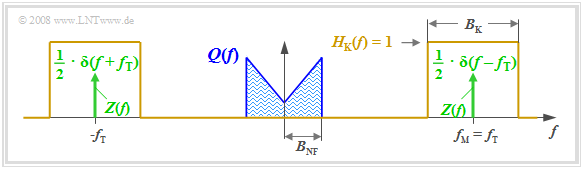Representation of the amplitude modulation in the frequency domain

The graphic clarifies the task, whereby the following simplifying assumptions are made:

• The drawn spectrum \$ Q (f) \$ is to be understood here schematically. It says that in \$ q (t) \$ only spectral components in the range \$ | f | ≤ B _ {\ rm NF} \$ are included. \$ Q (f) \$ could also be a line spectrum.
• Let the channel be ideal in a range of bandwidth \$ B _ {\ rm K} \$ around the frequency \$ f _ {\ rm M} \$, that is, \$ H _ {\ rm K} (f) = 1 \$ for \$ | f - f _ {\ rm M} | ≤ B _ {\ rm K} / 2.\$ Noise interference is not considered for the time being.
• Let the carrier signal be cosine \$ (\$ phase \$ ϕ_T = 0) \$ and have the amplitude \$ A _ {\ rm T} = 1 \$ (without unit). The carrier frequency \$ f _ {\ rm T} \$ is equal to the center frequency of the transmission band.
• The spectrum of the carrier signal \$ z (t) = \ cos (ω _ {\ rm T} · t) \$ is (shown in green in the graphic):
\$\$ Z (f) = {1} / {2} \ cdot \ delta (f + f _ {\ rm T}) + {1} / {2} \ cdot \ delta (f - f _ {\ rm T}) \ hspace {0.05cm}. \$\$

Anyone who is familiar with the laws of spectral transformation and especially with the convolution theorem can immediately give a solution for the spectrum \$ S (f) \$ of the modulator output signal:

\$\$ S (f) = Z (f) \ star Q (f) = 1/2 \ cdot \ delta (f + f _ {\ rm T}) \ star Q (f) +1/2 \ cdot \ delta ( f - f _ {\ rm T}) \ star Q (f) = 1/2 \ cdot Q (f + f _ {\ rm T}) + 1/2 \ cdot Q (f - f _ {\ rm T}) \ hspace {0.05cm}. \$\$

\$ \ text {Please note:} \$ This equation takes into account that the convolution of a shifted Dirac function \$ δ (x - x_0) \$ with any function \$ f (x) \$ die postponed function \$ f (x - x_0) \$ results.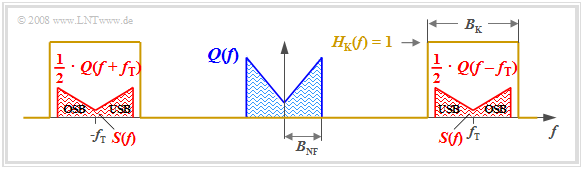Spectrum of the ZSB – AM without a carrier

The graphic shows the result. The following characteristics can be recognized:

• Due to the system-theoretical approach with positive and negative frequencies, \$ S (f) \$ is made up of two parts around \$ \ pm f _ {\ rm T} \$, each of which has the same form as \$ Q (f) \$.
• The factor \$ 1/2 \$ results from the carrier amplitude \$ A _ {\ rm T} = 1 \$. Thus \$ s (t = 0) = q (t = 0) \$, so that the integrals over their spectral functions \$ S (f) \$ or \$ Q (f) \$ must be the same.
• The channel bandwidth \$ B _ {\ rm K} \$ must be at least twice as large as the signal bandwidth \$ B _ {\ rm NF} \$, which has led to the name “double sideband amplitude modulation” (ZSB – AM).
• It should be noted that \$ B _ {\ rm NF} \$ and \$ B _ {\ rm K} \$ are absolute and not approximately equivalent bandwidths. The latter are defined by rectangles of equal area and are referred to in our tutorial with \$ Δf_q \$ or \$ Δf _ {\ rm K} \$.
• The spectral function \$ S (f) \$ does not contain any Dirac lines at the carrier frequency \$ (\ pm f _ {\ rm T}) \$. This is why this process is also called "ZSB – AM without carrier" designated.
• This is called the frequency components above the carrier frequency \$ f _ {\ rm T} \$ upper sideband (OSB), those below \$ f _ {\ rm T} \$ than that lower sideband (USB).

### Description in the time domain

With the nomenclature adapted to this problem, the convolution theorem reads:

\$\$ S (f) = Z (f) \ star Q (f) \ hspace {0.2cm} \ bullet \! \! - \! \! \! - \! \! \! - \! \! \ Circ \, \ hspace {0.2cm} s (t) = q (t) \ cdot z (t) = q (t) \ cdot \ cos (\ omega _ {\ rm T} \ cdot t + \ phi _ {\ rm T }) \ hspace {0.05cm}. \$\$

This result is still correct even if the restrictions \$ (\$ real-valued spectrum \$ Q (f) \$, carrier phase \$ ϕ _ {\ rm T} = 0) \$ are removed. In general, this results in a complex-valued spectral function \$ S (f) \$.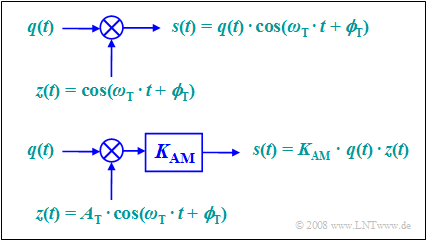Models of the ZSB – AM without carrier

According to this equation, two models can be given for the double sideband amplitude modulation. These are to be interpreted as follows:

• The first model directly describes the relationship given above, whereby the carrier \$ z (t) = \ cos (ω _ {\ rm T} t + ϕ _ {\ rm T}) \$ is assumed without a unit.
• The second model corresponds more to the physical conditions, since every signal also has a unit. If \$ q (t) \$ and \$ z (t) \$ are both voltages, the model also has a scaling with the modulator constant \$ K _ {\ rm AM} \$ (unit: \$ {\ rm V ^ {- 1}} \$ ) so that the output signal \$ s (t) \$ also represents a voltage curve.
• If you choose \$ K _ {\ rm AM} = 1 / A _ {\ rm T} \$, both models are the same. In the following we will always assume the simpler model.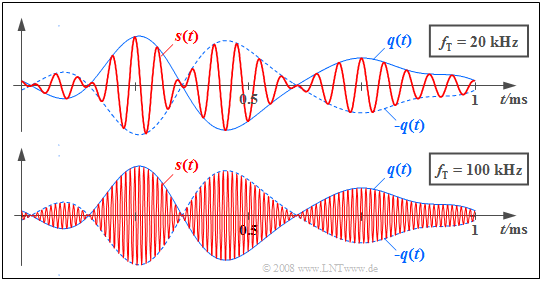Signal curves for the ZSB – AM without a carrier

\$ \ text {Example 1:} \$ The graphic shows the transmission signals \$ s (t) \$ for ZSB – AM for two different carrier frequencies in red.

• The source signal \$ q (t) \$, which is the same in both cases, with the bandwidth \$ B _ {\ rm NF} = 4 \ text {kHz} \$ is drawn continuously in blue and the signal \$ -q (t) \$ is dashed.
• The carrier signal \$ z (t) \$ has a cosine shape in both cases.
• The carrier frequency \$ f _ {\ rm T} = 20 \ text {kHz} \$ is used for the upper picture and \$ f _ {\ rm T} = 100 \ text {kHz} \$ for the lower picture.

### Ring modulator

The so-called “double sideband amplitude modulation with carrier suppression” offers one possibility for realizing the so-calledRing modulator, which is also called Double push-pull diode modulator is known. Below you can see the circuit on the left and a simple function diagram on the right.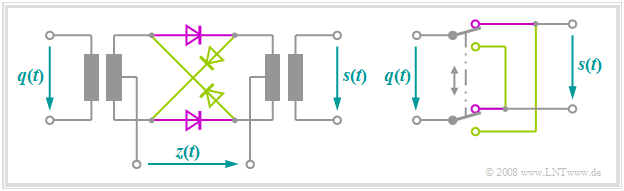Ring modulator to implement the AM assembly without a carrier

Without claiming to be exhaustive, the principle can be presented as follows:

• The amplitude of the harmonic carrier oscillation \$ z (t) \$ is much larger than the maximum value \$ q _ {\ rm max} \$ of the message signal \$ q (t) \$. Thus, all diodes are operated as switches.
• With a positive half-wave of the carrier oscillation \$ (z (t)> 0) \$, the two magenta-colored diodes conduct, while the olive-colored ones block. Without taking losses into account, \$ s (t) = q (t) \$ applies.
• In the case of a negative half-wave, the olive-colored diodes conduct and the diodes in the series branches block. As can be seen from the picture on the right, \$ s (t) = \ - q (t) \$ applies to this lower switch position.
• Because of the switch operation, the harmonic oscillation \$ z (t) \$ can also be replaced by a periodic square-wave signal of the same period:
\$\$ z _ {\ rm R} (t) = \ left \ {\ begin {array} {c} +1 \ -1 \ \ end {array} \ right. \ quad \ begin {array} {* { 10} c} {\ rm {f \ ddot {u} r}} \ {\ rm {f \ ddot {u} r}} \ \ end {array} \ begin {array} {* {20} c } {z (t)> 0,} \ {z (t) <0.} \ \ end {array} \$\$
• The modulated signal \$ s (t) \$ then results as the product of the message signal \$ q (t) \$ with this square-wave signal \$ z _ {\ rm R} (t) \$, while with an ideal ZSB-AM it is multiplied by a cosine signal.
• The carrier \$ z (t) \$ itself is not contained in the signal \$ s (t) \$. Since this is supplied via the center taps of the transformer, the induced voltages cancel each other out (“ZSB – AM without carrier”).

\$ \ text {Example 2:} \$ The mode of operation of the ring modulator will now be described again using exemplary signal curves. The carrier frequency is \$ f _ {\ rm T} = 10 \ text {kHz} \$.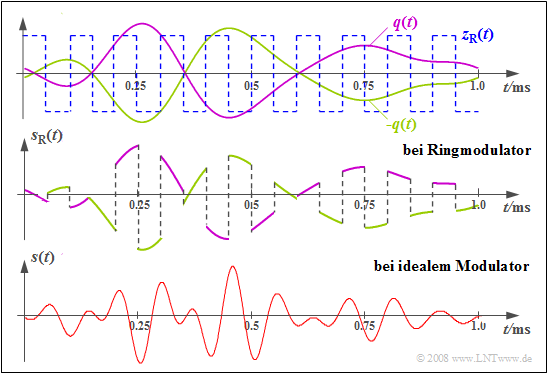Signals to illustrate the ring modulator
• The upper graphic shows the signals \$ q (t) \$ and \$ -q (t) \$ as magenta and olive-colored curves.
• For this purpose, the bipolar square-wave signal \$ z _ {\ rm R} (t) \$ is shown with a blue dashed line, which assumes the values ​​\$ ± 1 \$.
• The middle graphic shows the modulated signal of the ring modulator:
\$\$ s _ {\ rm RM} (t) = q (t) · z _ {\ rm R} (t). \$\$
• For comparison, the conventional AM signal is shown in the diagram below:
\$\$ s (t) = q (t) · \ cos (ω _ {\ rm T} · t). \$\$

You can see clear differences, but they can be easily compensated for:

• The Fourier series representation of the periodic square wave signal \$ z _ {\ rm R} (t) \$ is:
\$\$ z _ {\ rm R} (t) = \ frac {4} {\ pi} \ cdot \ cos (\ omega _ {\ rm T} \ cdot t) - \ frac {4} {3 \ pi} \ cdot \ cos (3 \ omega _ {\ rm T} \ cdot t) + \ frac {4} {5 \ pi} \ cdot \ cos (5 \ omega _ {\ rm T} \ cdot t) - \ text {... } \$\$
• The corresponding spectral function therefore consists of Dirac lines at \$ ± f _ {\ rm T}, ± 3f _ {\ rm T}, ± 5f _ {\ rm T} \$ etc. The convolution with \$ Q (f) \$ leads to the spectral function (the Index stands for "ring modulator"):
\$\$ S _ {\ rm RM} (f) = \ frac {2} {\ pi} \ cdot Q (f \ pm f _ {\ rm T}) - \ frac {2} {3 \ pi} \ cdot Q ( f \ pm 3f _ {\ rm T}) + \ frac {2} {5 \ pi} \ cdot Q (f \ pm 5f _ {\ rm T}) - \ text {...} \ hspace {0.05cm} \$ \$
• It can be seen from this that the well-known ZSB – AM spectrum can be obtained through a suitable band limitation \$ (\$ for example to \$ ± 2f _ {\ rm T}) \$ and an attenuation with \$ π / 4 ≈ 0.785 \$:
\$\$ S (f) = {1} / {2} \ cdot Q (f \ pm f _ {\ rm T}) \ hspace {0.05cm}. \$\$

With these considerations it must be taken into account that \$ B _ {\ rm NF} \ ll f _ {\ rm T} \$ can always be assumed.

### AM signals and spectra for a harmonic input signal

The special case, which is important for test purposes, should now be considered that not only the carrier signal \$ z (t) \$, but also the message signal \$ q (t) \$ to be modulated is a harmonic oscillation:

\$\$ \ begin {align *} q (t) & = A _ {\ rm N} \ cdot \ cos (\ omega _ {\ rm N} \ cdot t + \ phi _ {\ rm N}) \ hspace {0.05cm} , \ \ z (t) & = \ hspace {0.15cm} 1 \ hspace {0.13cm} \ cdot \ hspace {0.1cm} \ cos (\ omega _ {\ rm T} \ cdot t + \ phi _ {\ rm T}) \ hspace {0.05cm}. \ end {align *} \$\$

Please note: When describing modulation methods, the phase term with plus signs is taken into account in the above equations.

• Thus \$ ϕ _ {\ rm N} = - 90 ^ \ circ \$ stands for a sinusoidal input signal \$ q (t) \$ and \$ ϕ _ {\ rm T} = - 90 ^ \ circ \$ denotes a sinusoidal carrier signal \$ z (t) \$.
• The equation for the modulated signal is:
\$\$ s (t) = q (t) \ cdot z (t) = A _ {\ rm N} \ cdot \ cos (\ omega _ {\ rm N} t + \ phi _ {\ rm N}) \ cdot \ cos (\ omega _ {\ rm T} t + \ phi _ {\ rm T}) \ hspace {0.05cm}. \$\$

This equation can be transformed with the help of the addition theorem of trigonometry:

\$\$ s (t) = A _ {\ rm N} / {2} \ cdot \ cos \ big [(\ omega _ {\ rm T} + \ omega _ {\ rm N}) \ cdot t + \ phi _ {\ rm T} + \ phi _ {\ rm N} \ big] + A _ {\ rm N} / {2} \ cdot \ cos \ big [(\ omega _ {\ rm T} - \ omega _ {\ rm N}) \ cdot t + \ phi _ {\ rm T} - \ phi _ {\ rm N} \ big] \ hspace {0.05cm}. \$\$
• For cosine signals \$ (ϕ _ {\ rm T} = ϕ _ {\ rm N} = 0) \$ this equation is simplified to
\$\$ s (t) = {A _ {\ rm N}} / {2} \ cdot \ cos \ big [(\ omega _ {\ rm T} + \ omega _ {\ rm N}) \ cdot t \ big] + {A _ {\ rm N}} / {2} \ cdot \ cos \ big [(\ omega _ {\ rm T} - \ omega _ {\ rm N}) \ cdot t \ big] \ hspace {0.05cm}. \$ \$
• Fourier transformation leads to the spectral function:
\$\$ S (f) = {A _ {\ rm N}} / {4} \ cdot \ big [\ delta (f - f _ {\ rm T} - f _ {\ rm N}) + \ delta (f + f_ {\ rm T} + f _ {\ rm N}) \ big)] + {A _ {\ rm N}} / {4} \ cdot \ big [\ delta (f - f _ {\ rm T} + f _ {\ rm N}) + \ delta (f + f _ {\ rm T} - f _ {\ rm N}) \ big] \ hspace {0.05cm}. \$\$

This result, which one would also have come to via the convolution, says:

• The spectrum consists of four Dirac lines at the frequencies \$ ± (f _ {\ rm T} + f _ {\ rm N}) \$ and \$ ± (f _ {\ rm T} - f _ {\ rm N}) \$, where in both Expressions in brackets indicate the first Dirac function that indicates the positive frequency.
• The weights of all Dirac functions are equal and each is \$ A _ {\ rm N} / 4 \$. According to the theory, the sum of these weights - i.e. the integral over \$ S (f) \$ - is equal to the signal value \$ s (t = 0) = A _ {\ rm N} \$.
• The Dirac lines are also retained for \$ ϕ _ {\ rm T} ≠ 0 \$ and / or \$ ϕ _ {\ rm N} ≠ 0 \$ at the same frequencies. However, complex rotation factors must then be added to the weights \$ A _ {\ rm N} / 4 \$.

\$ \ text {Example 3:} \$ The following graphic shows the spectral functions \$ S (f) \$ for different values ​​of \$ ϕ _ {\ rm T} \$ or \$ ϕ _ {\ rm N} \$. The other parameters are \$ f _ {\ rm T} = 50 \ text {kHz} \$, \$ f _ {\ rm N} = 10 \ text {kHz} \$ and \$ A _ {\ rm N} = 4 \ text {V } \$ provided. The amounts of all Dirac lines are thus \$ A _ {\ rm N} / 4 = 1 \ text {V} \$.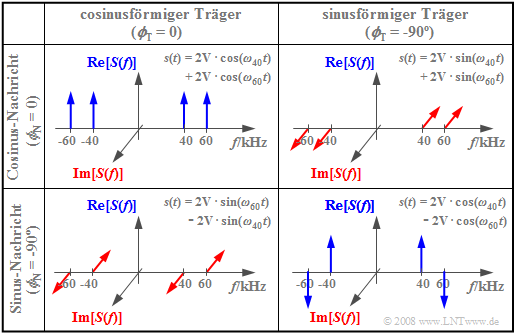Exemplary spectra of the ZSB – AM
• The upper left picture shows the case just discussed: Both the carrier and the message signal are cosine-shaped. The amplitude-modulated signal \$ s (t) \$ is thus composed of two cosine oscillations with \$ ω_ {60} = 2 π · 60 \ text {kHz} \$ and \$ ω_ {40} = 2 π · 40 \ text {kHz} \$ .
• In the three other constellations, at least one of the signals \$ q (t) \$ or \$ z (t) \$ is sinusoidal, so that always \$ s (0) = 0 \$. Thus, in these spectra, the sum of the four pulse weights is zero.
• The lower right figure describes \$ s (t) = A _ {\ rm N} · \ sin (ω _ {\ rm N} t) · \ sin (ω _ {\ rm T} t) \$. The multiplication of two odd functions results in the even function \$ s (t) \$ and thus a real spectrum \$ S (f) \$. In contrast, the other two constellations each lead to imaginary spectral functions.

### ZSB amplitude modulation with carrier

The following graphic shows how to get from the “AM assembly without carrier” to the more well-known variant “AM assembly with carrier”. This has the advantage that the demodulator can be implemented much more simply and cheaply by a simple measure at the transmitter.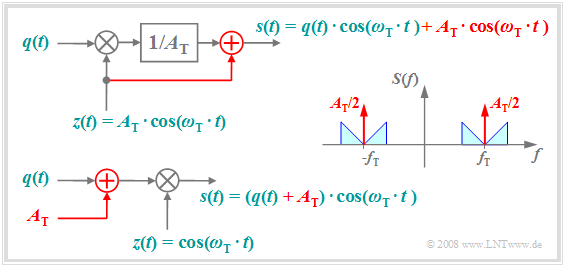Models of the ZSB – AM with carrier

The graphic is to be interpreted as follows:

• The upper illustration shows the physical model of the “AM assembly with carrier”, with changes compared to the “AM assembly without carrier” being highlighted in red.
• The carrier signal \$ z (t) = A _ {\ rm T} · \ cos (ω _ {\ rm T} · t) \$ is added to the signal \$ s (t) \$, which has two additional Dirac functions in the spectrum at \$ ± f_ {\ rm T} \$ causes, each with the momentum weight \$ A _ {\ rm T} / 2 \$.
• Adding the DC signal \$ A _ {\ rm T} \$ to the source signal and then multiplying it by the dimensionless carrier \$ z (t) \$ according to the diagram below results in the same signal \$ s (t) \$ and the same spectrum \$ S (f ) \$ as above.
• The second representation is therefore equivalent to the above model. In both cases, the carrier phase is set to \$ ϕ _ {\ rm T} = 0 \$ for reasons of a simplified representation.

\$ \ text {Example 4:} \$ Die Double sideband amplitude modulation with carrier still finds its main application in radio transmission today

• Long wave \$ (\$ frequency range \$ \ text {30 kHz} \$ ... \$ \ text {300 kHz}) \$,
• Medium wave \$ (\$ frequency range \$ \ text {300 kHz} \$ ... \$ \ text {3 MHz}) \$,
• Shortwave \$ (\$ frequency range \$ \ text {3 MHz} \$ ... \$ \ text {30 MHz}) \$.

However, these frequencies are being released more and more for digital applications, for example for Digital video broadcast (DVB).

An application of Double sideband amplitude modulation without a carrier there is, for example, FM stereo broadcasting:

• Here the difference signal between the two stereo channels is amplitude-modulated at \$ \ text {39 kHz} \$ carrierless.
• Then the sum signal of the two channels \$ (\$ each in the range \$ \ text {30 Hz} \$ ... \$ \ text {15 kHz}) \$, a subcarrier at \$ \ text {19 kHz} \$ and the difference signal are combined and frequency modulated.

\$ \ text {Example 5:} \$ The following signal curves are intended to further clarify the principle of the "AM assembly with carrier".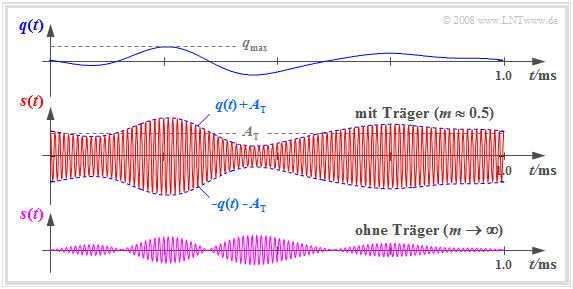Signal curves for the AM assembly with carrier
• Above you can see a section of the source signal \$ q (t) \$ limited to frequencies \$ \ vert f \ vert \ le 4 \ text {kHz} \$.
• \$ s (t) \$ is obtained by adding the constant component \$ A _ {\ rm T} \$ to \$ q (t) \$ and the signal sum with the carrier signal \$ z (t) \$ of the frequency \$ f _ {\ rm T} = 100 \ text {kHz} \$ multiplied.
• For comparison, the transmission signal of the “ZSB – AM without carrier” is shown below.

A comparison of these signal curves shows:

• By adding the constant component \$ A _ {\ rm T} \$ it was achieved that the message signal \$ q (t) \$ can now be recognized in the envelope of \$ s (t) \$.
• In this way, envelope demodulation can be used, which is easier and cheaper to implement than coherent synchronous demodulation.
• A prerequisite for using the envelope demodulator is a degree of modulation \$ m <1 \$. This is defined as follows:
\$\$ m = \ frac {q _ {\ rm max}} {A _ {\ rm T}} \ hspace {0.3cm} {\ rm with} \ hspace {0.3cm} q _ {\ rm max} = \ max_ {t } \ hspace {0.05cm} \ vert q (t) \ vert \ hspace {0.05cm}. \$\$
• The advantage of a simpler demodulator, however, has to be bought at the cost of a significantly higher transmission power, since the carrier's contribution to performance cannot be used for demodulation.
• It is also important to ensure that the source signal does not contain any direct component, as this would be covered by the carrier. In the case of voice and music signals, however, this is not a major restriction.

### Description of the ZSB-AM by the analytical signal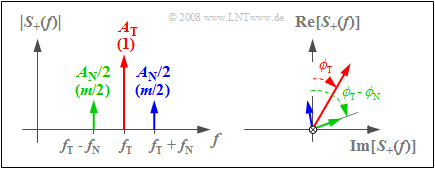Spectrum of the analytical signal in two different forms of representation

In the following, the spectrum \$ S _ + (f) \$ of the analytical signal is usually given instead of the actual, physical spectrum \$ S (f) \$ to simplify graphics.

As an example we consider a "ZSB-AM with carrier" and the following signals:

\$\$ \ begin {align *} s (t) & = \ left (q (t) + A _ {\ rm T} \ right) \ cdot \ cos (\ omega _ {\ rm T} \ cdot t + \ phi_ { \ rm T}) \ hspace {0.05cm}, \ \ q (t) & = A _ {\ rm N} \ cdot \ cos (\ omega _ {\ rm N} \ cdot t + \ phi _ {\ rm N }) \ hspace {0.05cm}. \ end {align *} \$\$

Then the corresponding analytical signal reads:

\$\$ s _ + (t) = A _ {\ rm T} \ cdot {\ rm e} ^ {{\ rm j} \ hspace {0.03cm} \ cdot \ hspace {0.01cm} (\ omega _ {\ rm T} \ cdot \ hspace {0.02cm} t \ hspace {0.05cm} + \ hspace {0.05cm} \ phi _ {\ rm T})} + \ frac {A _ {\ rm N}} {2} \ cdot {\ rm e} ^ {{\ rm j} \ hspace {0.03cm} \ cdot \ hspace {0.01cm} ((\ omega _ {\ rm T} \ hspace {0.05cm} + \ hspace {0.05cm} \ omega _ {\ rm N}) \ hspace {0.02cm} \ cdot \ hspace {0.02cm} t \ hspace {0.05cm} + \ hspace {0.05cm} \ phi _ {\ rm T} + \ phi _ {\ rm N})} + \ frac {A _ {\ rm N}} {2} \ cdot {\ rm e} ^ {{\ rm j} \ hspace {0.03cm} \ cdot \ hspace {0.01cm} ((\ omega _ {\ rm T} \ hspace {0.05cm} - \ hspace {0.05cm} \ omega _ {\ rm N}) \ hspace {0.02cm} \ cdot \ hspace {0.02cm} t \ hspace {0.05cm} + \ hspace {0.05cm} \ phi_ {\ rm T} - \ phi _ {\ rm N})} \ hspace {0.05cm}. \$\$

The associated spectral function \$ S _ + (f) \$ consists of three Dirac lines, each with complex weights according to the graphic:

• The sketch on the left shows the amount \$ | S _ + (f) | \$, where \$ A _ {\ rm T} \$ indicates the weight of the girder and \$ A _ {\ rm N} / 2 \$ the weights of OSB (upper sideband) and USB (lower sideband).
• The values ​​normalized to \$ A _ {\ rm T} \$ are in brackets. Since \$ q _ {\ rm max} = A _ {\ rm N} \$ applies here, one obtains with the degree of modulation \$ m = A _ {\ rm N} / A _ {\ rm T} \$ as normalized weights of the upper and lower sidebands \$ m / \$ 2.
• The right sketch gives a view in the direction of the frequency axis and shows the phase angles of carrier \$ (ϕ _ {\ rm T}) \$, USB \$ (ϕ _ {\ rm T} - ϕ _ {\ rm N}) \$ and OSB \$ (ϕ_ {\ rm T} + ϕ _ {\ rm N}) \$.

### Amplitude modulation through quadratic characteristic

Non-linearities are usually undesirable and disruptive in communications engineering. As explained in the chapter Nonlinear Distortions of the book "Linear Time-Invariant Systems", they lead to

• the superposition principle is no longer applicable,
• the transmission behavior depends on the size of the input signal, and
• the distortions are of a non-linear nature and are therefore irreversible.

A general form non-linearity

\$\$ y (t) = c_0 + c_1 \ cdot x (t) + c_2 \ cdot x ^ 2 (t) + c_3 \ cdot x ^ 3 (t) + \ text {...} \$\$

but can also be used to implement an AM assembly. On condition that

• only the coefficients \$ c_1 \$ and \$ c_2 \$ are available, and
• the input signal \$ x (t) = q (t) + z (t) \$ is applied,

one obtains for the output signal of the non-linearity:

\$\$ y (t) = c_1 \ cdot q (t) + c_1 \ cdot z (t) + c_2 \ cdot q ^ 2 (t) + 2 \ cdot c_2 \ cdot q (t) \ cdot z (t) + c_2 \ cdot z ^ 2 (t) \ hspace {0.05cm}. \$\$

The first, the third and the last part are - seen spectrally - at \$ | f | ≤ 2 · B _ {\ rm NF} \$ or \$ | f | = 2 · f _ {\ rm T} \$.

If one removes these signal components by a bandpass and takes into account \$ z (t) = A _ {\ rm T} · \ cos (ω _ {\ rm T} · t) \$, one obtains the equation typical for “ZSB – AM with carrier”
(only the second and fourth term):

\$\$ s (t) = c_1 \ cdot A _ {\ rm T} \ cdot \ cos (\ omega _ {\ rm T} \ cdot t) + 2 \ cdot c_2 \ cdot A _ {\ rm T} \ cdot q (t ) \ cdot \ cos (\ omega _ {\ rm T} \ cdot t) \ hspace {0.05cm}. \$\$

With this type of implementation, the degree of modulation can be changed using the coefficients \$ c_1 \$ and \$ c_2 \$:

\$\$ m = \ frac {2 \ cdot c_2 \ cdot q _ {\ rm max}} {c_1} \ hspace {0.05cm}. \$\$

A diode and the field effect transistor have such a square characteristic with good approximation and can be used to implement an AM assembly. Cubic components \$ (c_3 ≠ 0) \$ and nonlinearities of higher order lead to (large) nonlinear distortions.

### Exercises for the chapter

Exercise 2.1: AM assembly with cosine? Or with sine?

Exercise 2.1Z: ZSB-AM without / with carrier

Exercise 2.2: Degree of Modulation

Exercise 2.2Z: performance analysis

Exercise 2.3: AM assembly

Exercise 2.3Z: ZSB through non-linearity